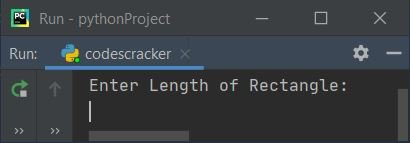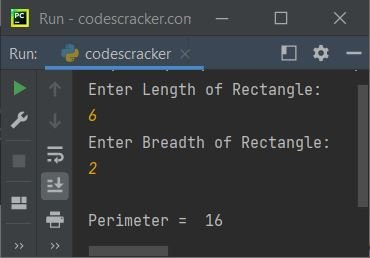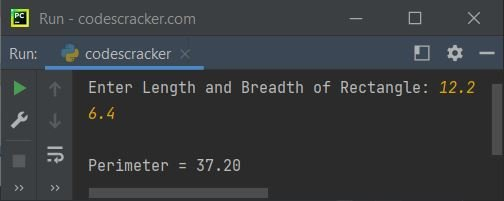# Python Program to Find Perimeter of Rectangle

In this article, we've created some programs in Python, to find and print perimeter of a rectangle based on the length and breadth entered by user at run-time. Here are the list of approaches used to do the task:

• Find Perimeter of Rectangle without using Function
• Using user-defined Function
• Using class and object

Before creating these programs, let me remind you about the formula used here.

### Formula to Find Perimeter of Rectangle

To find perimeter value of a rectangle, use following formula:

```per = len+bre+len+bre
= (2*len)+(2*bre)
= 2*(len+bre)```

Here per indicates to perimeter, len indicates to length and bre indicates to breadth of rectangle. You can use any one from these three formulae. The third one is better to go with.

## Find Perimeter of Rectangle without Function

To calculate perimeter of rectangle in Python, you have to ask from user to enter the length and breadth value of rectangle whose perimeter you want to find out. The question is, write a Python program to find perimeter of rectangle. Here is its answer:

```print("Enter Length of Rectangle: ")
l = int(input())
b = int(input())
p = 2*(l+b)
print("\nPerimeter = ", p)```

Here is the initial output produced by this Python program:Now supply inputs say 6 as length and 2 as breadth, press `ENTER` key to find and print perimeter as shown in the snapshot given below:#### Modified Version of Previous Program

This program uses end= to skip an automatic printing of newline using print(). The {:.2f} is used to print the value inside format() method upto two decimal places only.

```print("Enter Length and Breadth of Rectangle: ", end="")
l = float(input())
b = float(input())
print("\nPerimeter = {:.2f}".format(2*(l+b)))```

Here is its sample run with user input, 12.2 as length and 6.4 as breadth of rectangle:## Find Perimeter of Rectangle using Function

This program uses a user-defined function named findPeri() to do the same task as of previous program. The function takes two arguments. First argument refers to length and second refers to breadth of rectangle. The function returns perimeter value. Therefore, perimeter value gets initialized to res and the value of it gets printed as perimeter of rectangle. That's it.

```def findPeri(a, b):
p = 2*(a+b)
return p

print("Enter Length and Breadth of Rectangle: ", end="")
l = float(input())
b = float(input())

res = findPeri(l, b)
print("\nPerimeter = {:.2f}".format(res))```

This program produces exactly same output as of previous program.

## Find Perimeter of Rectangle using Class

This is the last program on finding the perimeter of a rectangle, using class and object, an object-oriented feature of Python.

```class CodesCracker:
def findPeri(self, a, b):
p = 2*(a+b)
return p

print("Enter Length and Breadth of Rectangle: ", end="")
l = float(input())
b = float(input())

ob = CodesCracker()
res = ob.findPeri(l, b)
print("\nPerimeter = {:.2f}".format(res))```

In above program, an object ob gets created of class CodesCracker(). So that it can be used to access the member function of the class say findPeri() using dot (.) operator. Rest of the things works similar to a normal function.

#### Same Program in Other Languages

Python Online Test

« Previous Program Next Program »